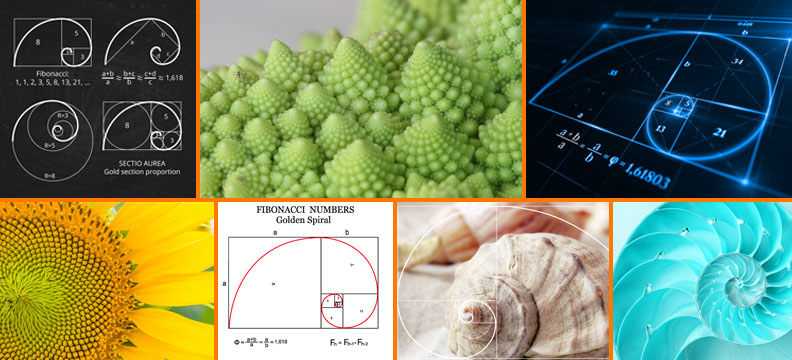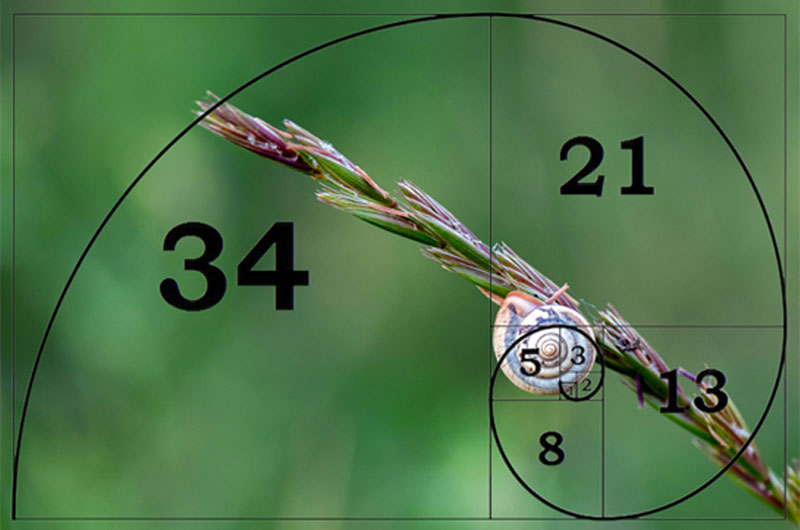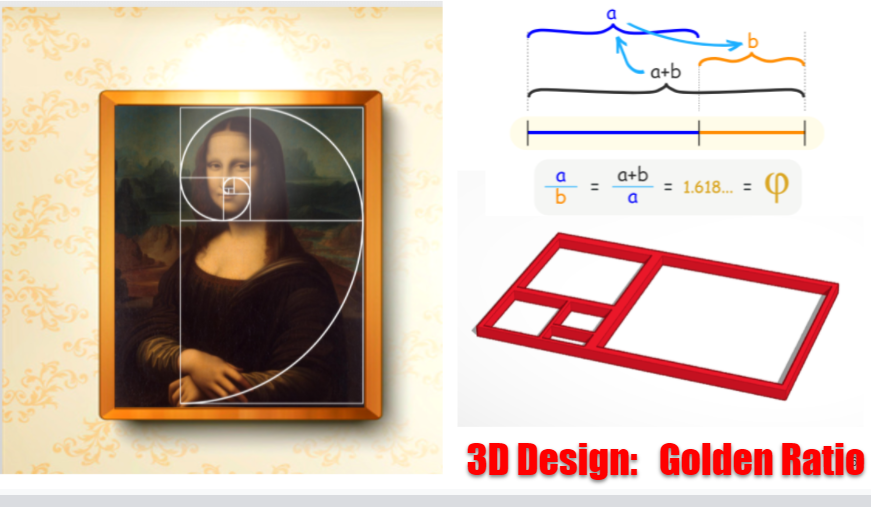Friday, August 27, 2021 - 10:34

The Fibonacci Sequence

in math and nature.Such a beautiful and interesting concept—nature inspired balance.

Many sources claim it was first discovered or "invented" by Leonardo Fibonacci. The Italian mathematician, originally known as Leonardo of Pisa. But Leonardo of Pisa did not actually discover the sequence — Ancient Sanskrit texts that used the Hindu-Arabic numeral system first mention it, and those predate Leonardo of Pisa by centuries. In 1202 Leonardo of Pisa published the massive tome "Liber Abaci," a mathematics "cookbook for how to do calculations. In one place in the book, Leonardo of Pisa introduces the sequence with a problem involving rabbits. The problem goes as follows: Start with a male and a female rabbit. After a month, they mature and produce a litter with another male and female rabbit. A month later, those rabbits reproduce and out comes — you guessed it — another male and female, who also can mate after a month. (Ignore the wildly improbable biology here.) After a year, how many rabbits would you have? The answer, it turns out, is 144 — and the formula used to get to that answer is what's now known as the Fibonacci sequence, and after a few thin paragraphs on breeding rabbits, Leonardo of Pisa never mentioned the sequence again.

In the 19th century the term Fibonacci sequence was coined by the French mathematician Edouard Lucas, and scientists began to discover such sequences in nature.

This amazing phenomenon is everywhere we look! We observe that many of the natural things follow the Fibonacci sequence. It appears in biological settings such as branching in trees, phyllotaxis (the arrangement of leaves on a stem), the fruit sprouts of a pineapple, the flowering of an artichoke, an uncurling fern, and the arrangement of a pinecone’s bracts and so much more!In simple math terms the Fibonacci Sequence is a set of numbers that starts with a one or a zero, followed by a one, and proceeds based on the rule that each number (called a Fibonacci number) is equal to the sum of the preceding two numbers. But it’s much more than that.

The Fibonacci sequence exhibits a certain numerical pattern, this pattern turns out to have an interest and importance far beyond what was first imagined. It can be used to model or describe an amazing variety of phenomena, in mathematics and science, art and nature. The mathematical ideas the Fibonacci sequence, such as the golden ratio, spirals and self- similar curves, have long been appreciated for their charm and beauty, but no one can really explain why they are echoed so clearly in the world of art and nature.Our STEM:IT High School course has a lesson that utilizes the Golden Ratio - Math with the topic of Ratios, this math lesson incorporates art! Students have the opportunity to do a 3D print project with this. Neat, huh?